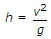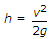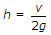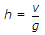# Civil Engineering - Hydraulics - Discussion

### Discussion :: Hydraulics - Section 1 (Q.No.15)

15.

Critical depth (h) of a channel, is

 [A].[B].[C].[D].Explanation:

No answer description available for this question.

 Javed said: (Jul 2, 2015) As it depends on froudes number. For critical flow froudes number is one and the depth corresponding to critical flow is called critical depth. Froudes number = Velocity/Root of height*velocity.

 Divakar said: (Apr 28, 2016) What's v2/g? Please explain.

 Vishu said: (Aug 6, 2016) It is possible only for the rectangular channel.

 Sreesha said: (Sep 4, 2016) As we know, that v is directly proportional to √ g*h according to Froude's law.

 Ravi said: (Nov 18, 2016) Froud number equals to 1 for critical so (V/(√gh)):1.

 Bapugouda said: (Dec 24, 2016) Please, anyone give me the explanation of the answer.

 Abhishek Anand said: (Feb 12, 2017) The depth of water in a channel when vel of flow is critical or when the specific energy is min is called critical depth of the channel. Critical vel of flow is the vel at which sp.energy is minimum. Specific energy -> E=h+(v^2/2g). For minimization, dE/dh =0. On putting v=Q/A. We get , 1=v^2/gh. So, V= (gh)^1/2 or sqrt of gh. Which is critical velocity; So, critical depth= v^2/g.

 Vaibhav Patle said: (Feb 27, 2017) No, the right answer is v2/2g.

 Sandip Buktare said: (Mar 2, 2017) For critical depth = v/root of height * gravity. 1 = v/root of height * gravity, The root of height * gravity = v, Taking square of both side. h * g = v^2 h = v^/g.

 Santhosh C said: (May 1, 2017) V in the above equation is critical velocity. That is velocity with respect to the critical depth of flow.

 Santhosh C said: (May 1, 2017) Critical velocity Vc = root of g * hc(Critical depth of flow).

 Rohit said: (Jun 17, 2017) V^2/2g is the right answer.

 Nitesh Saini said: (Nov 16, 2017) A is correct answer. Reason behind that v^2/2g is a term used in specific energy not for critical depth i.e. E= h+v^2/2g = specific energy. But critical depth is that depth at which specific energy is minimum so we have to differentiate above equation w.r.t h ... And then term comes dE/dh= 1+ q^2/2g(-2/h^3)=0 ... And simplify this equating we will get h= q^2/g .... i.e. (av)^2/g... i.e. v^2/g. I hope you will understand what i m saying good luck friends for your future.

 Kajal Ncp said: (Jul 31, 2018) Thanks @Nitesh.

 Thundir said: (Dec 20, 2018) E = y + v^2/2g is specific energy. Here, v^2/2g is KINETIC energy and "y" is POTENTIAL energy. So, the given answer is correct.

 Anurag said: (Jan 30, 2019) I think [q^2/g]^[1/3].

 Amare Yihunie said: (Feb 2, 2019) For critical flow type specific energy = yc + v2/2g. for rectangular channel = Ec = 1.5 yc. 1.5 yc = yc + v2/2g, 0.5 yc = v2/2g, yc = v2/g.

 Priyanka Shah said: (Mar 6, 2019) Thank you @Nitesh.

 Prassy said: (Apr 6, 2020) hc=( q2/g)^1/3. Taking cube on both sides hc^3= q2/g. q=Q/b= A*V/b= (b*h*V)/b= (h*v), hc^3= (hc * V)^2/g, hc= V2/g.

 Sudip Timalsena said: (Jan 22, 2021) Thank you @Prassy.

 Sanjay Panchal said: (Feb 25, 2021) Thanks.